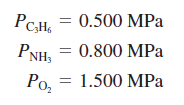# Problem: An important process for the production of acrylonitrile (C3H3N) is given by the following equation:2C3H6(g) + 2NH3(g) + 3O2(g) → 2C3H3N(g) + 6H2O(g)A 150.-L reactor is charged to the following partial pressures at 25°C:What mass of acrylonitrile can be produced from this mixture (MPa = 10 6 Pa)?

###### FREE Expert Solution
• According to the ideal gas equation, pressure is directly proportional to # of moles. (as P increases, n increases at constant V and T  (Avogadro's Law))
• Therefore, whichever has the least amount of P will be considered as the limiting reactant. (C3H6 at 0.500 MPa). MPa here is a unit of P
• Moles of the limiting reactant can be solved using the ideal gas equation and the conditions provided
• Mass of acrylonitrile can be solved using this moles of limiting reactant and the balanced equation provided.
97% (101 ratings)###### Problem Details

An important process for the production of acrylonitrile (C3H3N) is given by the following equation:

2C3H6(g) + 2NH3(g) + 3O2(g) → 2C3H3N(g) + 6H2O(g)

A 150.-L reactor is charged to the following partial pressures at 25°C:What mass of acrylonitrile can be produced from this mixture (MPa = 10 6 Pa)?